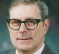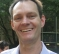## Looking for an exotic channel coderStarted by 1 year ago11 replieslatest reply 1 year ago123 views

Hi everyone. I fear that what I am looking for might not exist, but I'll still ask - you never know.

I need to transmit quantized measurement values over a noisy channel. Let's say for the sake of this example that my values are quantized to 5bits ranging from -16,...+15. If a single bit fails in the transmission, the maximum error in value would be 16 (sign bit failing: e.g. 0b10000=-16 -> 0b00000 = 0, or 0b01111 = 15 -> 0b11111 = -1), the minimum error in value would be 1 if the LSB fails (e.q. 0b00000 = 0 -> 0b00001 = 1).

Bandwidth is scarce, so I'll only introduce the slightest forward error correction possible and only if I absolutely need to. I can accept bit errors - I only care for value errors.

Example:

0b00111 = 7 -> 0b01000 = 8

This example has 4 bit errors, but the value only changes by 1.

Here's the question:

Is there a method to encode binary numbers or a vector of a few binary numbers into a bit stream such that bit errors cause minimal changes in the VALUE of the binary numbers? Small added redundancy is accepted. Anybody?

[ - ]Do a search on the phrase "Gray code". It's a method of encoding positive binary numbers so that each two consecutive numbers differ in their encoding by a single bit.  This can't work with the sign bit, but if you only encode 31 different consecutive numbers 0 to 30, assign those same codes to -15 to 15.

[ - ]**edit**

I first thought, gray code would solve my problem, but it doesn't. While it ensures that consecutive coded numbers differ by only one bit, it does not ensure, that changing any of the other bits keeps the error minimal. Since the code is cyclic, the max and min value also differ by only one bit. If this bit fails, the error will still be 2^N-1.

**end edit - old answer below**

oh my! I have used Gray coding a lot in the past - usually to assign bit sequences to higher order modulation schemes - to make sure only one bit drops when mistaking one symbol for the closest neighbour - I even thought about gray coding while writing my question above - but I dismissed it thinking it only prevents too many bit errors, but of course it works the other way around: Causing only one bit error in a Gray coded signal will take you to a neighbouring symbol - in my case a neighbouring number. Thanks! Should have been obvious...

[ - ]Can you accept that some values are deemed invalid, or do you need all of them ?

Is there any profile in the type of noise you have ?

Do the channel work differently (less noisy) when many or few changes in bits ?

Are there any seuquence roules, like value can only change up or down by 2 ?

Do you have a clear idea (timer) about when the "word" starts in the recieving end ?

[ - ]Can you accept that some values are deemed invalid, or do you need all of them ?

** edit **

-> after thinking twice: I can accept that some values are invalid. This is equal to adding redundancy or having less possible values than 2^Nbits. I can accept using 6bits for 32 values if that keeps the error small.

** edit end **

[deleted: -> I need all of them]

Is there any profile in the type of noise you have ?

-> unknown - assuming AWGN

Do the channel work differently (less noisy) when many or few changes in bits ?

-> no

Are there any seuquence roules, like value can only change up or down by 2 ?

-> no, the values already are the result of data compression, so they are basically white - meaning they can take on any value independantly of each other

Do you have a clear idea (timer) about when the "word" starts in the recieving end ?

-> yes, synchronization is not the problem

[ - ]You tolerate bit errors but not denary(decimal value) yet the denary value is transmitted as binary.

In principle you can give priority to bits based on their weight. Or - in theory only - transmit denary system or constellation states. Otherwise it doesn't make much sense to me.

[ - ]"You tolerate bit errors but not denary(decimal value) yet the denary value is transmitted as binary."

Almost true. I do accept errors in the denary value. I just want to keep them as small as possible. In my 5bit example above, the error in the denary value caused by a single bit error ranges between 1 and 16. I would much prefer a coding scheme that limits the effect of a single bit error on the denary value. Something like a guarantee that the denary value will not change by more than 5 (or 1 or 6 or N :).

I would also accept the addition of few redundant bits if that's what it takes. Some sort of "forward error impact reduction" instead of FEC.

[ - ]Since you compress the bit stream, each bit error in the compressed stream may affect several bits in the binary word, and those bits, though contiguous, could be anywhere in the word, and hence any amount of error in the denary word.  Because of this compression, I suggest you focus on rms error rather than maximum error.  In this case you can compute (with much effort) or simulate the translation from compressed bit error density to rms (or mean) denary error.

In summary, there is no way to code the binary word to minimize the denary error if you are compressing the binary stream, so you should instead try to minimize rms or mean error instead of peak error.

I am not a DSP expert, so take this as a concept to vet rather than a fact.

[ - ]Sorry - I wasn't clear enough - I do compress the stream but not as in zip or lha, but as in lossy ADPCM - so the 5bit values are still samples representing some sort of analog-like value. Only - to restore the original - you have to scale and filter (usually integrate) them heavily.

So bit errors have a direct influence on the sample value and do not affect other bits.

[ - ]